# Category Archives: Models

## Kinetic Limits on Engineering Agonist Drugs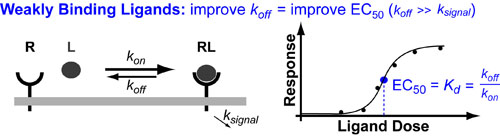There are many drugs that act as agonists ligands (L) which means they “turn on” their target receptor (RL) so that it induces its normal down-stream signalling. Examples of such drugs include: growth hormones, insulin, steroids and G-protein coupled Recetor(GCPR) ligands such as morphine (opiods), neurotransmitters and scent/aroma compounds. In general you can improve the potency (EC50) of these drugs by improve their binding dissociation constant (Kd) for their receptor (for more detail see post on the Hill Equation).

## Binding #3: Competitive Inhibition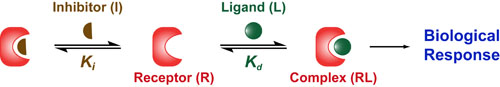While many drugs act like agonists and “turn on” their target/receptor (see posts on Hill Equation and Agonist Limits), many more drugs act like antagonists and “turn off” their target/receptor. One of the most common mechanisms by which drugs “turn off” a target receptor (R) is by blocking the binding of its natural ligand (L). This mechanism is called competitive inhibition and the antagonist is known as an inhibitor (I). Here there are two dissociation constants:the Kd which describes the strength of the interaction between R and L and the Ki which describes the strength of the interaction between R and I.

## Binding #2: The Michaelis-Menton Equation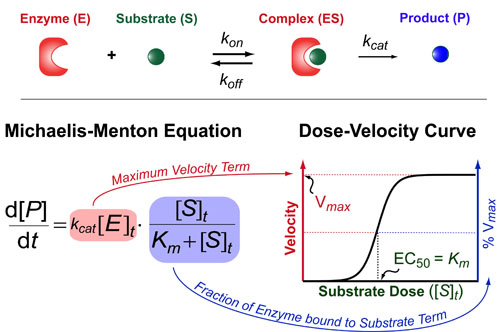The Michaelis-Menton Equation has a very similar form to the Hill Equation but the key difference is that it deals with enzyme rates not ligand/receptor or drug/target interactions per se. Basically, it describes how fast an enzyme (E) makes its product (P) as a function of the total concentration of substrate ([S]t). This rate of production formation (d[P]/dt) is proportional to the kcat and the amount of complex ([ES]) which is exact what the Michaelis-Menton equation models. The Michaelis-constant (Km = (koff+kcat) / kon) describes how tightly the substrate binds the enzyme and the kcat is a rate-constant that describes how quickly the enzyme can make the product. Here,brackets denote concentrations and a t subscript indicates “total concentrations.”

## Binding#1: Understanding the Hill Equation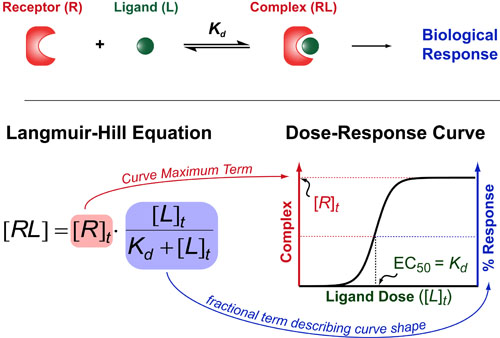If you want to understand how drugs work/behave (or just how 2 molecules interact with each other) then you need to understand one of the oldest and most useful theories in biochemistry: the Hill Equation. The Hill Equation mathematically models how Ligands(L)/Drugs interaction with their Receptors(R)/targets and generally assumes that the amount of complex that forms (RL) is proportional to some biological response . Here the Kd represents the “dissociation constant” (which is a measure of the strength of the interaction between R and L) and brackets denote concentrations/dosages (with a t subscript indicating total concentrations).

## How do Experimentalists use Mathematical Models?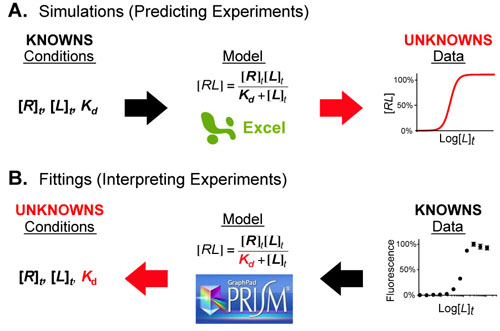Figure 1.  Mathematical models act as a bridge between the conditions of an experimental system and its behavior (the data). When a portion of either is unknown you can combine the knowns (black arrows) and calculate the unknowns (red arrows) using simple software packages such as Excel or Prism.

Mathematical models are equations (or systems of equations) that allow one the connect the conditions of a system/experiment with its predicted behavior/experimental data. Figure 1 shows an example of this where the Langmuir-Hill equation (in the center) connects the conditions (left) of a fluorescence binding assay with the dose-response curve (right) that results from conducting the experiment.

## Understanding Ligand-Receptor Dose Response Curves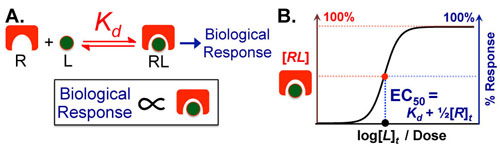Figure 1 A.
Most Ligand (L)–Receptor (R) dose–response curves are characterized by formation of a complex followed by biological effect. The underlying assumption is that biological effect is proportional to the amount of complex that forms. B. Assuming the biological effect and amount of complex formed are proportional, dose–response curves can be envisioned on two equivalent axes: amount complex (in red) and percentage biological response (in blue). The key to intuitively understanding these curves is to understand the Effective Concentration 50% or EC50 which tells you the dose of ligand necessary to elicit a 50% response. The EC50 is also known as the ligand’s potency.

Biological phenomena are often caused by the binding of a ligand (L (e.g. drug, hormone, etc.)) to a target receptor (R), which induces a biological response (Figure 1A). Typically, the biological response is proportional to the amount of ligand-receptor complex (RL) that forms (Figure 1A); as a result, the dose–response curve can have two equivalent y-axes: amount of complex (in red) or biological response (in blue). These curves have a sigmoidal (S-like) shape, where low doses of ligand have no effect and high doses plateau at maximal (100%) complex formation/biological response (Figure 1B).